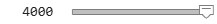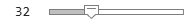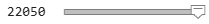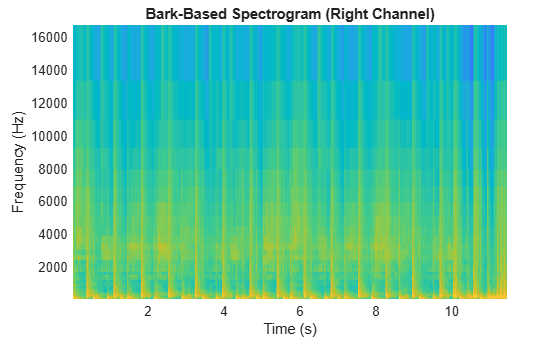Documentation

# designAuditoryFilterBank

Design auditory filter bank

## Syntax

``filterBank = designAuditoryFilterBank(fs)``
``filterBank = designAuditoryFilterBank(fs,Name,Value)``
``[filterBank,Fc,BW] = designAuditoryFilterBank(___)``

## Description

example

````filterBank = designAuditoryFilterBank(fs)` returns a frequency-domain auditory filter bank, `filterBank`.```

example

````filterBank = designAuditoryFilterBank(fs,Name,Value)` specifies options using one or more `Name,Value` pair arguments.```
````[filterBank,Fc,BW] = designAuditoryFilterBank(___)` returns the center frequency and bandwidth of each filter in the filter bank. You can use this output syntax with any of the previous input syntaxes.```

## Examples

collapse all

Call `designAuditoryFilterBank` with a specified sample rate to design the default auditory filter bank.

```fs = 44.1e3; fb = designAuditoryFilterBank(fs);```

The default filter bank consists of 32 triangular bandpass filters spaced evenly on the mel scale between 0 and `fs`/2 Hz.

`numBands = size(fb,1)`
```numBands = 32 ```

`designAuditoryFilterBank` is intended for use in frequency-domain filtering for a one-sided spectrum. By default, `designAuditoryFilterBank` assumes a 1024-point DFT, so it returns a half-sided frequency-domain filter bank with 513 points.

`numPoints = size(fb,2)`
```numPoints = 513 ```

Read in audio and convert it to a one-sided power spectrum.

```[audioIn,fs] = audioread("Laughter-16-8-mono-4secs.wav"); win = hamming(1024,"periodic"); noverlap = 512; fftLength = 1024; [~,F,t,PowerSpectrum] = spectrogram(audioIn,win,noverlap,fftLength,"power","onesided",fs);```

Design a mel-based auditory filter bank. Plot the filter bank.

```numBands =32; range = [0,4000]; normalization ="bandwidth"; [fb,cf] = designAuditoryFilterBank(fs, ... "FFTLength",fftLength, ... "NumBands",numBands, ... "FrequencyRange",range, ... "Normalization",normalization); plot(F,fb.') grid on title("Mel Filter Bank") xlabel("Frequency (Hz)")```To apply frequency domain filtering, perform a matrix multiplication of the filter bank and the power spectrogram.

`X = fb*PowerSpectrum;`

Visualize the power-per-band in dB.

```XdB = 10*log10(X); surf(t,cf,XdB,"EdgeColor","none"); xlabel("Time (s)") ylabel("Frequency (Hz)") zlabel("Power (dB)") view([45,60]) title('Mel-Based Spectrogram') axis tight```Read in audio and convert it to a one-sided power spectrum.

```[audioIn,fs] = audioread("RockDrums-44p1-stereo-11secs.mp3"); win = hann(round(0.03*fs),"periodic"); noverlap = round(0.02*fs); fftLength = 2048; [~,F,t,PowerSpectrumLeft] = spectrogram(audioIn(:,1),win,noverlap,fftLength,"power","onesided",fs); [~,~,~,PowerSpectrumRight] = spectrogram(audioIn(:,2),win,noverlap,fftLength,"power","onesided",fs);```

Design a Bark-based auditory filter bank. Plot the filter bank.

```numBands =32; range = [0,22050]; normalization ="area"; [fb,cf] = designAuditoryFilterBank(fs, ... "FrequencyScale","bark", ... "FFTLength",fftLength, ... "NumBands",numBands, ... "FrequencyRange",range, ... "Normalization",normalization); plot(F,fb.'); grid on title("Bark Filter Bank") xlabel("Frequency (Hz)")```To apply frequency domain filtering, perform a matrix multiplication of the filter bank and the left and right power spectrograms.

```XL = fb*PowerSpectrumLeft; XR = fb*PowerSpectrumRight;```

Visualize the power-per-band in dB.

```XLdB = 10*log10(XL); XRdB = 10*log10(XR); surf(t,cf,XLdB,"EdgeColor","none"); xlabel("Time (s)") ylabel("Frequency (Hz)") view([0,90]) title("Bark-Based Spectrogram (Left Channel)") axis tight``````surf(t,cf,XRdB,"EdgeColor","none"); xlabel("Time (s)") ylabel("Frequency (Hz)") view([0,90]) title("Bark-Based Spectrogram (Right Channel)") axis tight```Read in audio and convert it to a one-sided power spectrum.

```[audioIn,fs] = audioread("NoisySpeech-16-22p5-mono-5secs.wav"); win = hann(round(0.04*fs),"periodic"); noverlap = round(0.02*fs); fftLength = 1024; [~,F,t,PowerSpectrum] = spectrogram(audioIn,win,noverlap,fftLength,"power","onesided",fs);```

Design an ERB-based auditory filter bank. Plot the filter bank.

```numBands =32; range = [0,11025]; normalization ="bandwidth"; [fb,cf] = designAuditoryFilterBank(fs, ... "FrequencyScale","erb", ... "FFTLength",fftLength, ... "NumBands",numBands, ... "FrequencyRange",range, ... "Normalization",normalization); plot(F,fb.'); grid on title("ERB Filter Bank") xlabel("Frequency (Hz)")```To apply frequency-domain filtering, perform a matrix multiplication of the filter bank and the power spectrogram.

`X = fb*PowerSpectrum;`

Visualize the power-per-band in dB.

```XdB = 10*log10(X); surf(t,cf,XdB,"EdgeColor","none"); xlabel("Time (s)") ylabel("Frequency (Hz)") view([0,90]) title("ERB-Based Spectrogram") axis tight```## Input Arguments

collapse all

Sample rate of filter design in Hz, specified as a positive scalar.

Data Types: `single` | `double`

### Name-Value Pair Arguments

Specify optional comma-separated pairs of `Name,Value` arguments. `Name` is the argument name and `Value` is the corresponding value. `Name` must appear inside quotes. You can specify several name and value pair arguments in any order as `Name1,Value1,...,NameN,ValueN`.

Example: `"FrequencyScale","mel"`

Frequency scale used to design the auditory filter bank, specified as the comma-separated pair consisting of `'FrequencyScale'` and `"mel"`, `"bark"`, or `"erb"`.

Data Types: `char` | `string`

Number of points used to calculate the DFT, specified as the comma-separated pair consisting of `'FFTLength'` and a positive integer.

Data Types: `single` | `double`

Number of bandpass filters, specified as the comma-separated pair consisting of `'NumBands'` and a positive integer. The default number of bandpass filters depends on the `FrequencyScale`:

• If `FrequencyScale` is set to `"bark"` or `"mel"`, then `NumBands` defaults to `32`.

• If `FrequencyScale` is set to `"erb"`, then `NumBands` defaults to `ceil(hz2erb(FrequencyRange(2))-hz2erb(FrequencyRange(1)))`.

Data Types: `single` | `double`

Frequency range over which to design auditory filter bank in Hz, specified as the comma-separated pair consisting of `'FrequencyRange'` and a two-element row vector of monotonically increasing values in the range [0, `fs`/2].

Data Types: `single` | `double`

Normalization technique used on the weights of the filter bank:

• `"bandwidth"` –– The weights of each bandpass filter are normalized by the corresponding bandwidth of the filter.

• `"area"` –– The weights of each bandpass filter are normalized by the corresponding area of the bandpass filter.

• `"none"` –– The weights of the filters are not normalized.

Data Types: `char` | `string`

## Output Arguments

collapse all

Auditory filter bank, returned as an M-by-N matrix, where M is the number of bands (`NumBands`), and N is the number of frequency points of a one-sided spectrum (`ceil(FFTLength/2)`).

Data Types: `double`

Center frequencies of bandpass filters in Hz, returned as a row vector with `NumBands` elements.

Data Types: `double`

Bandwidth of bandpass filters in Hz, returned as a row vector with `NumBands` elements.

Data Types: `double`

## Algorithms

The mel filter bank is designed as half-overlapped triangles equally spaced on the mel scale. 

The Bark filter bank is designed as half-overlapped triangles equally spaced on the Bark scale. 

The ERB filter bank is designed as gammatone filters  whose center frequencies are equally spaced on the ERB scale. 

 O'Shaghnessy, Douglas. Speech Communication: Human and Machine. Reading, MA: Addison-Wesley Publishing Company, 1987.

 Traunmüller, Hartmut. "Analytical Expressions for the Tonotopic Sensory Scale." Journal of the Acoustical Society of America. Vol. 88, Issue 1, 1990, pp. 97–100.

 Glasberg, Brian R., and Brian C. J. Moore. "Derivation of Auditory Filter Shapes from Notched-Noise Data." Hearing Research. Vol. 47, Issues 1–2, 1990, pp. 103–138.

 Slaney, Malcolm. "An Efficient Implementation of the Patterson-Holdworth Auditory Filter Bank." Apple Computer Technical Report 35, 1993.

##### SupportGet trial now